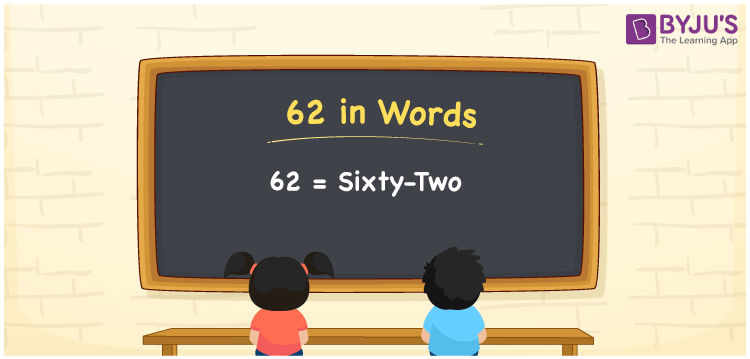# 62 in Words

62 in words is written as “Sixty-two”. 62 is a two-digit number that has two place values, ones and tens. It represents a count, quantity or value. To represent 62 in words, we should know how to read and write it in English since it is the most commonly used language. Also, learning to spell numbers in words is necessary to utilise in real-life situations. For example, Ravi saved Sixty-two Rupees out of shopping today. Learn how to write 62 in words in this article.

 62 in words Sixty-two Sixty-two in Numbers 62

## 62 in English Words

We generally write numbers in words using the English alphabet. Thus, we can read 62 in English as “Sixty-two”.## How to Write 62 in Words?

To convert 62 in words, we need to check the place value of its digits. Since 62 is a two-digit number, thus, place value of 6 is tens and 2 is ones. The order of place values of digits in a number is in accordance with the Indian numbering system, such as:

• Ones
• Tens
• Hundreds
• Thousands
• Ten-thousands
• Hundred-thousands or Lakhs

This can be done as follows.

 Tens Ones 6 2

This can be written as:

6 × Ten + 2 × One

= 6 × 10 + 2 × 1

= 60 + 2

= 62

= Sixty-two

Thus, 62 in words is written as Sixty-two.

62 is a natural number that precedes 63 and succeeds 61.

62 in words – Sixty-two

Is 62 an odd number? – No

Is 62 an even number? – Yes

Is 62 a perfect square number? – No

Is 62 a perfect cube number? – No

Is 62 a prime number? – No

Is 62 a composite number? – Yes

## Frequently Asked Questions on 62 in Words

Q1

### How do you write 62 in English?

We can write 62 in English words as “Sixty-two”.
Q2

### What is 362 in English?

362 is written as Three hundred and Sixty-two in English.
Q3

### Is 62 an even number or an odd number?

62 is an even number since it is completely divisible by 2.